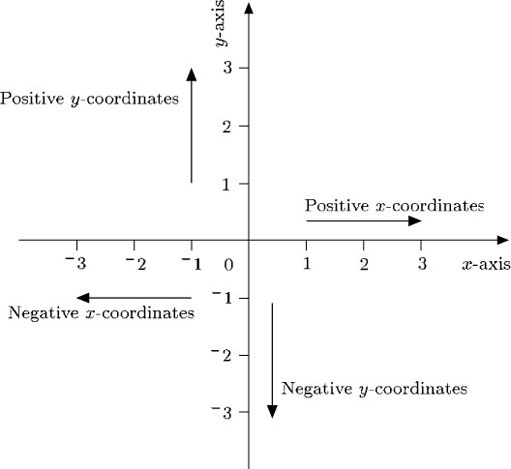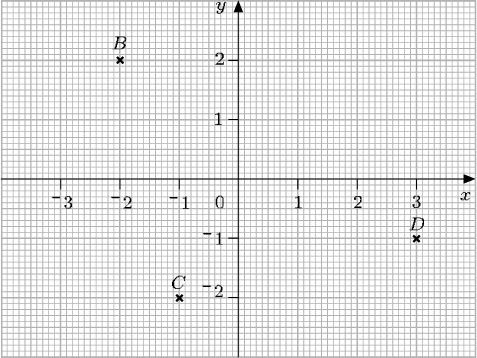Science, Maths & Technology

### Become an OU studentDiagrams, charts and graphs

Start this free course now. Just create an account and sign in. Enrol and complete the course for a free statement of participation or digital badge if available.

# 3.2 Negative coordinates

Up to now only those points with positive or zero coordinates have been considered. But the system can be made to cope with points involving negative coordinates, such as (−2, 3) or (−2, −3). Just as a number line can be extended to deal with negative numbers, the x-axis and y-axis can be extended to deal with negative coordinates.In this way, if a point is to the left of the origin, its x-coordinate is negative, and if it is below the origin, its y-coordinate is negative. The origin itself is (0, 0).

## Example 10

Write down the coordinates of the points B, C and D. Remember that the x-coordinate is given first, and the y-coordinate is given second.